## Example Questions

← Previous 1 3 4 5

### Example Question #11 : Quadratic Equations

What is the sum of the two solutions of the equation x2 + 5x – 24 = 0?

-24/5

3

-5

24/5

-24

-5

Explanation:

First you must find the solutions to the equation. This can be done either by using the quadratic formula or by simply finding two numbers whose sum is 5 and whose product is -24 and factoring the equation into (x + 8)(x – 3) = 0. The solutions to the equation are therefore -8 and 3, giving a sum of -5.

### Example Question #12 : Quadratic Equations

The height of a ball (in feet) after it is thrown in the air is given by the expression

s(t) = –t2 + 4t

where t is time in seconds. The ball is thrown from ground level at t = 0. How many seconds will pass before the ball reaches the ground again?

10

2

6

8

4

4

Explanation:

Notice that when the ball is at ground level, the height is zero. Setting (t) equal to zero and solving for t will then give the times when the ball is at the ground.

–t2 + 4t =0

t(4 – t) = 0

t = 0, t = 4

The ball returns to the ground after 4 seconds.

### Example Question #3 : How To Find The Solution To A Quadratic Equation A1

Two positive consecutive multiples of 3 have a product of 180.  What is the sum of the two numbers?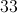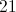Explanation:

Define varables as= the first number and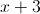= the second number.  The product of the numbers is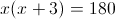.  Solve the resulting quadratic equation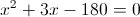by factoring and setting each factor to zero.  The numbers are 12 and 15 and the sum is 27.

### Example Question #4 : How To Find The Solution To A Quadratic Equation A1

Given the equation: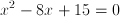.

What is the product of the solutions of the quadratic equation?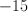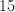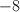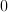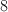Explanation:

We are initially presented with a quadratic equation,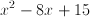. To begin we must factor this equation.

The multiples of 15 are (15 and 1) and (3 and 5). The only multiples that add or subtract toare 3 and 5. Hence we use these as our binomial numbers.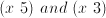. We must now decide on the signs. Because we need to add or subtract 5 and 3 to get to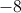, both signs must be negative:.

From this point we need to switch gears to find solutions to the equation. What numbers would make this equation equal 0?

At this point split the equation into two parts.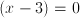and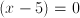and solve.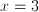and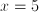. Both of these numbers inserted into the original equation will produce a result of 0.

Now the question itself is asking for the product of the solutions to the equation, or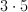, which equals 15, therefore 15 is our answer.

### Example Question #5 : How To Find The Solution To A Quadratic Equation A1

If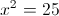and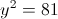, what is the greatest value that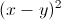can have?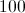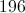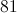Explanation:

Solving foryields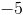and. Solving for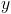yields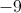and.

The greatest difference between these two numbers is 14, and 14 squared is 196.

### Example Question #6 : How To Find The Solution To A Quadratic Equation A1

Two positive consecutive multiples of three have a product of 108.  What is their sum?Explanation:

Let= 1st number

and= 2nd number

So the equation to solve becomes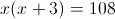or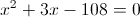We factor to solve the quadratic equation to get 9 and 12 and their sum is 21.

### Example Question #7 : How To Find The Solution To A Quadratic Equation A1

Two consecutive positive odd numbers have a product of 99,  What is the sum of the two numbers?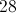Explanation:

Let= 1st odd number and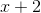= 2nd odd number.

So the equation to solve becomes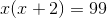or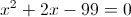Solving the quadratic equation by factoring gives 9 and 11, so the sum is 20

### Example Question #3 : How To Find The Solution To A Quadratic Equation A1

When asked how many home runs he hit in a season, Pablo Sanchez responded with, "If you square the number of home runs and subtract 50 times the number of home runs, it is equivalent to 50." How many home runs has Pablo hit?

73

49

1

51

1

51

Explanation:

We can generate an equation for the number of home runs he has hit, x : x2 - 50x = 50.  Reordering this, we get : x2 - 50x - 50 = 0.  Using the quadratic equation: x = (-b± √(b2-4ac)) / (2a). In this case, a = 1, b = -50, c = -50.  Plugging in these values, we obtain the simplified equation, x = (50±51.96)/2.  Therefore, x = 50.98, -0.98.  Because it doesn't make sense to have a negative number of home runs, x = 50.98, which rounds up to 51 home runs.

### Example Question #1 : How To Find The Solution To A Quadratic Equation A1

If (x + a)(x + b) = x2  9x + 18, what are the values for a and b?

a = 3, b = 6

a = 3, b = 6

a = 6, b =3

a = 3, b = 6

a = 3, b = 6

a = 3, b = 6

Explanation:

a = 3, b = 6.  The sum of a and b have to be equal to 9, and they have to multiply together to get +18. If a = 3 and b = 6, (3) + (6) = (9) and (3)(6) = 18.

### Example Question #1 : How To Find The Solution To A Quadratic Equation A1

Which of the following is the closest approximate solution for x where 11x– 7x – 8 = 0?

4

19/22

27/22

–29/22

7/11